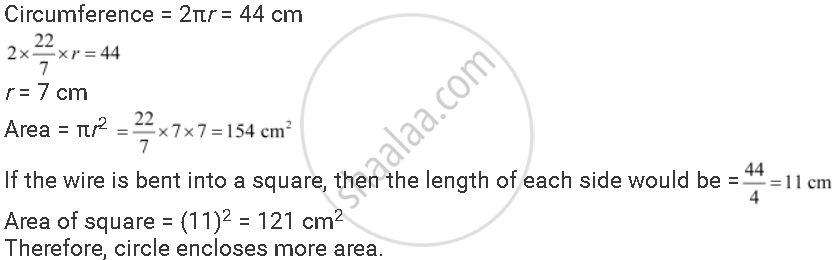Share

# Shazli Took a Wire of Length 44 Cm and Bent It into the Shape of a Circle. Find the Radius of that Circle. Also Find Its Area. If the Same Wire is Bent into the Shape of a Square, What Will Be the Length of Each of Its Sides? Which Figure Encloses More Area, the Circle Or the Square? - CBSE Class 7 - Mathematics

ConceptCircle Circumference of a Circle

#### Question

Shazli took a wire of length 44 cm and bent it into the shape of a circle. Find the radius of that circle. Also find its area. If the same wire is bent into the shape of a square, what will be the length of each of its sides? Which figure encloses more area, the circle or the square? (Take pi = 22/7)

#### SolutionIs there an error in this question or solution?

#### APPEARS IN

NCERT Solution for Mathematics for Class 7 (2018 to Current)
Chapter 11: Perimeter and Area
Ex. 11.30 | Q: 9 | Page no. 223
Solution Shazli Took a Wire of Length 44 Cm and Bent It into the Shape of a Circle. Find the Radius of that Circle. Also Find Its Area. If the Same Wire is Bent into the Shape of a Square, What Will Be the Length of Each of Its Sides? Which Figure Encloses More Area, the Circle Or the Square? Concept: Circle - Circumference of a Circle.
S